Anzeige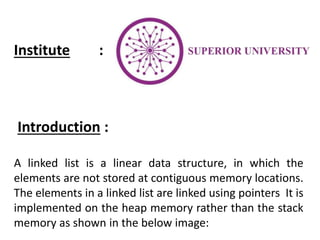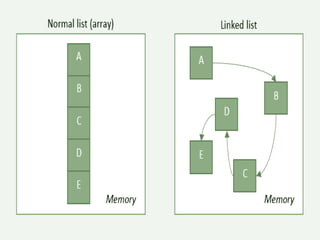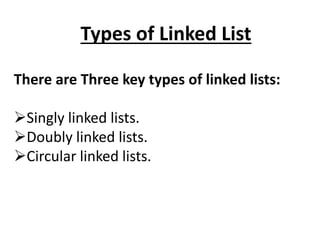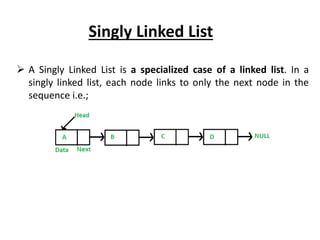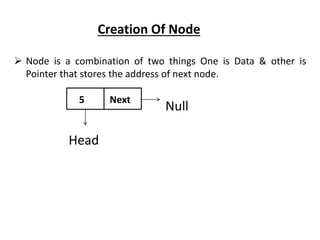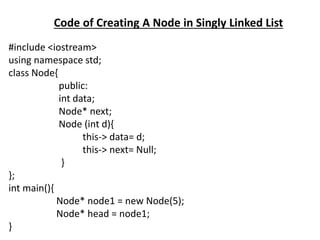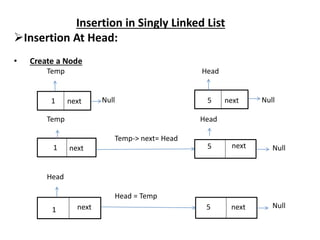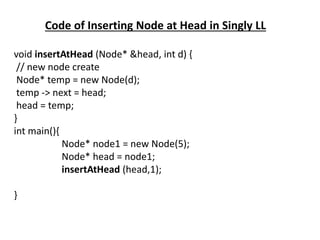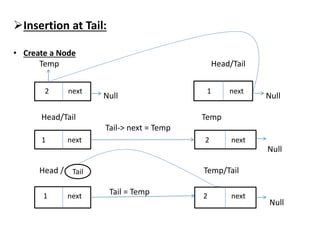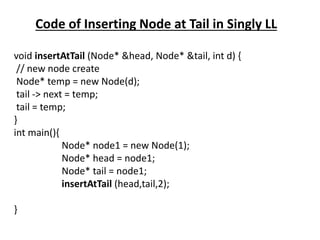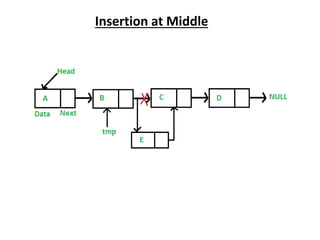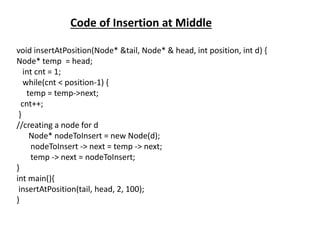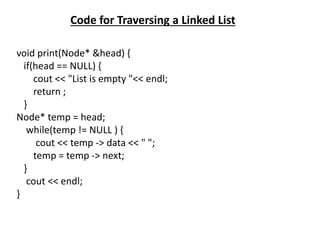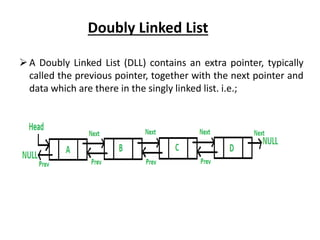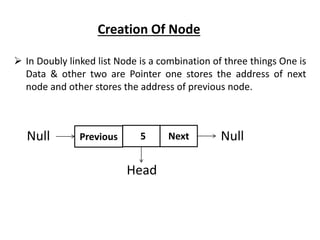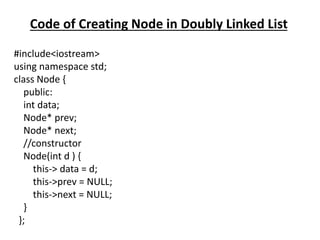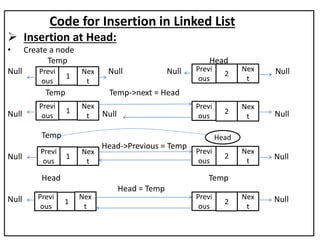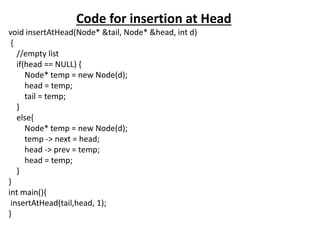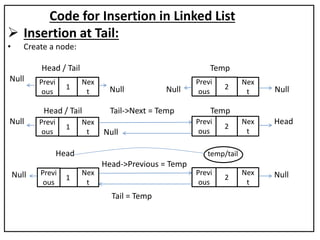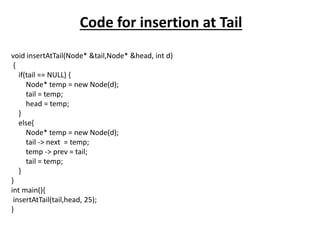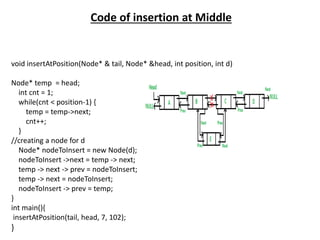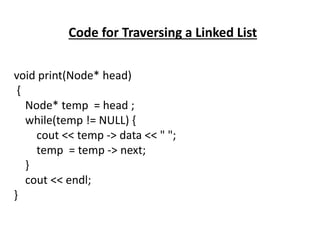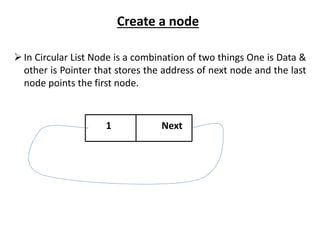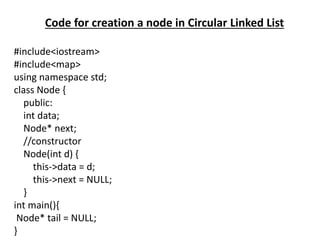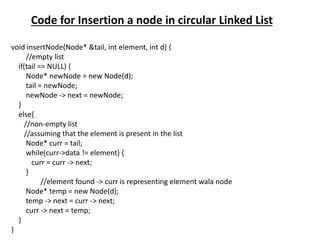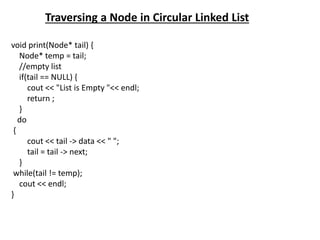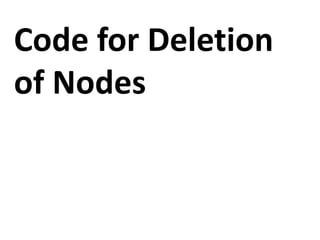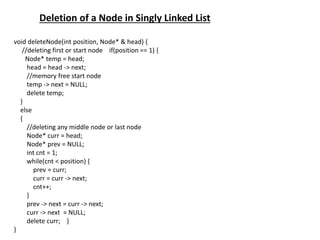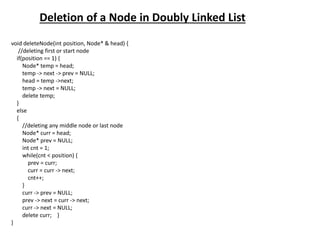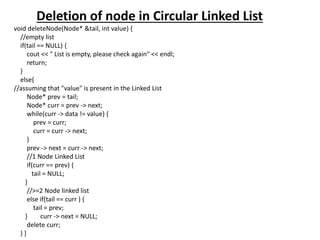1 von 36
Anzeige

### DSA(1).pptx

1. Program : CS(computer Science) Semester : 3rd Course : Data Structures & Algorithms Presented By : Daniyal,Moazzam,Ayyan Presented To : Prof. Asad Bilal Topic : Linked List
2. Institute : Introduction : A linked list is a linear data structure, in which the elements are not stored at contiguous memory locations. The elements in a linked list are linked using pointers It is implemented on the heap memory rather than the stack memory as shown in the below image:
4. Singly Linked List  A Singly Linked List is a specialized case of a linked list. In a singly linked list, each node links to only the next node in the sequence i.e.;
5. Creation Of Node  Node is a combination of two things One is Data & other is Pointer that stores the address of next node. Null Head 5 Next
6. Code of Creating A Node in Singly Linked List #include <iostream> using namespace std; class Node{ public: int data; Node* next; Node (int d){ this-> data= d; this-> next= Null; } }; int main(){ Node* node1 = new Node(5); Node* head = node1; }
7. Insertion in Singly Linked List Insertion At Head: • Create a Node Temp Head Null Null Temp Head Temp-> next= Head Null Head Head = Temp Null 1 5 1 5 1 5 next next next next next next
8. Code of Inserting Node at Head in Singly LL void insertAtHead (Node* &head, int d) { // new node create Node* temp = new Node(d); temp -> next = head; head = temp; } int main(){ Node* node1 = new Node(5); Node* head = node1; insertAtHead (head,1); }
9. Insertion at Tail: • Create a Node Temp Head/Tail Null Null Head/Tail Temp Tail-> next = Temp Null Head / Temp/Tail Tail = Temp Null 2 next 1 next 1 next Tail 2 next 1 next 2 next
10. Code of Inserting Node at Tail in Singly LL void insertAtTail (Node* &head, Node* &tail, int d) { // new node create Node* temp = new Node(d); tail -> next = temp; tail = temp; } int main(){ Node* node1 = new Node(1); Node* head = node1; Node* tail = node1; insertAtTail (head,tail,2); }
11. Insertion at Middle
12. Code of Insertion at Middle void insertAtPosition(Node* &tail, Node* & head, int position, int d) { Node* temp = head; int cnt = 1; while(cnt < position-1) { temp = temp->next; cnt++; } //creating a node for d Node* nodeToInsert = new Node(d); nodeToInsert -> next = temp -> next; temp -> next = nodeToInsert; } int main(){ insertAtPosition(tail, head, 2, 100); }
13. Code for Traversing a Linked List void print(Node* &head) { if(head == NULL) { cout << "List is empty "<< endl; return ; } Node* temp = head; while(temp != NULL ) { cout << temp -> data << " "; temp = temp -> next; } cout << endl; }
14. Doubly Linked List A Doubly Linked List (DLL) contains an extra pointer, typically called the previous pointer, together with the next pointer and data which are there in the singly linked list. i.e.;
15. Creation Of Node  In Doubly linked list Node is a combination of three things One is Data & other two are Pointer one stores the address of next node and other stores the address of previous node. Null Null Head 5 Next Previous
16. Code of Creating Node in Doubly Linked List #include<iostream> using namespace std; class Node { public: int data; Node* prev; Node* next; //constructor Node(int d ) { this-> data = d; this->prev = NULL; this->next = NULL; } };
17. Code for Insertion in Linked List  Insertion at Head: • Create a node Temp Head Null Null Null Null Temp Temp->next = Head Null Null Null Temp Head->Previous = Temp Null Null Head Temp Head = Temp Null Null Previ ous 1 Nex t Previ ous 2 Nex t Previ ous 1 Nex t Nex t 1 1 Previ ous Previ ous Nex t Previ ous Previ ous Previ ous 2 2 2 Nex t Nex t Nex t Head
18. Code for insertion at Head void insertAtHead(Node* &tail, Node* &head, int d) { //empty list if(head == NULL) { Node* temp = new Node(d); head = temp; tail = temp; } else{ Node* temp = new Node(d); temp -> next = head; head -> prev = temp; head = temp; } } int main(){ insertAtHead(tail,head, 1); }
19. Code for Insertion in Linked List  Insertion at Tail: • Create a node: Head / Tail Temp Null Null Null Null Head / Tail Tail->Next = Temp Temp Null Head Null Head Head->Previous = Temp Null Null Tail = Temp Previ ous 1 Nex t Previ ous 2 Nex t Previ ous 1 Nex t Nex t 1 Previ ous Previ ous Previ ous 2 2 Nex t Nex t temp/tail
20. Code for insertion at Tail void insertAtTail(Node* &tail,Node* &head, int d) { if(tail == NULL) { Node* temp = new Node(d); tail = temp; head = temp; } else{ Node* temp = new Node(d); tail -> next = temp; temp -> prev = tail; tail = temp; } } int main(){ insertAtTail(tail,head, 25); }
21. Code of insertion at Middle void insertAtPosition(Node* & tail, Node* &head, int position, int d) Node* temp = head; int cnt = 1; while(cnt < position-1) { temp = temp->next; cnt++; } //creating a node for d Node* nodeToInsert = new Node(d); nodeToInsert ->next = temp -> next; temp -> next -> prev = nodeToInsert; temp -> next = nodeToInsert; nodeToInsert -> prev = temp; } int main(){ insertAtPosition(tail, head, 7, 102); }
22. Code for Traversing a Linked List void print(Node* head) { Node* temp = head ; while(temp != NULL) { cout << temp -> data << " "; temp = temp -> next; } cout << endl; }
23. Circular Linked List The circular linked list is a linked list where all nodes are connected to form a circle. In a circular linked list, the first node and the last node are connected to each other which forms a circle. There is no NULL at the end.
24. Create a node In Circular List Node is a combination of two things One is Data & other is Pointer that stores the address of next node and the last node points the first node. 1 Next
25. Code for creation a node in Circular Linked List #include<iostream> #include<map> using namespace std; class Node { public: int data; Node* next; //constructor Node(int d) { this->data = d; this->next = NULL; } int main(){ Node* tail = NULL; }
26. Code for Insertion a node in circular Linked List void insertNode(Node* &tail, int element, int d) { //empty list if(tail == NULL) { Node* newNode = new Node(d); tail = newNode; newNode -> next = newNode; } else{ //non-empty list //assuming that the element is present in the list Node* curr = tail; while(curr->data != element) { curr = curr -> next; } //element found -> curr is representing element wala node Node* temp = new Node(d); temp -> next = curr -> next; curr -> next = temp; } }
27. Traversing a Node in Circular Linked List void print(Node* tail) { Node* temp = tail; //empty list if(tail == NULL) { cout << "List is Empty "<< endl; return ; } do { cout << tail -> data << " "; tail = tail -> next; } while(tail != temp); cout << endl; }
28. Code for Deletion of Nodes
29. Deletion of a Node in Singly Linked List void deleteNode(int position, Node* & head) { //deleting first or start node if(position == 1) { Node* temp = head; head = head -> next; //memory free start node temp -> next = NULL; delete temp; } else { //deleting any middle node or last node Node* curr = head; Node* prev = NULL; int cnt = 1; while(cnt < position) { prev = curr; curr = curr -> next; cnt++; } prev -> next = curr -> next; curr -> next = NULL; delete curr; } }
30. Deletion of a Node in Doubly Linked List void deleteNode(int position, Node* & head) { //deleting first or start node if(position == 1) { Node* temp = head; temp -> next -> prev = NULL; head = temp ->next; temp -> next = NULL; delete temp; } else { //deleting any middle node or last node Node* curr = head; Node* prev = NULL; int cnt = 1; while(cnt < position) { prev = curr; curr = curr -> next; cnt++; } curr -> prev = NULL; prev -> next = curr -> next; curr -> next = NULL; delete curr; } }
31. Deletion of node in Circular Linked List void deleteNode(Node* &tail, int value) { //empty list if(tail == NULL) { cout << " List is empty, please check again" << endl; return; } else{ //assuming that "value" is present in the Linked List Node* prev = tail; Node* curr = prev -> next; while(curr -> data != value) { prev = curr; curr = curr -> next; } prev -> next = curr -> next; //1 Node Linked List if(curr == prev) { tail = NULL; } //>=2 Node linked list else if(tail == curr ) { tail = prev; } curr -> next = NULL; delete curr; } }
32. So, We have our Questions for You People ?
Anzeige